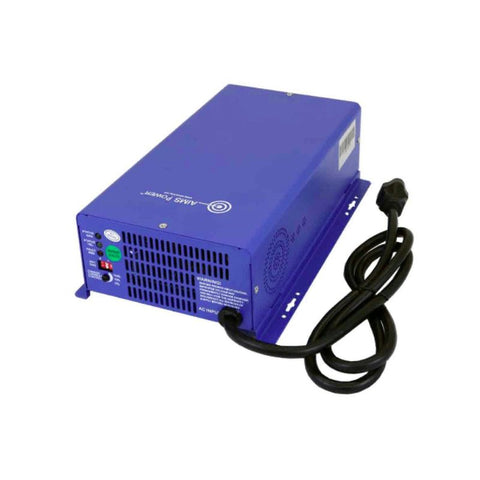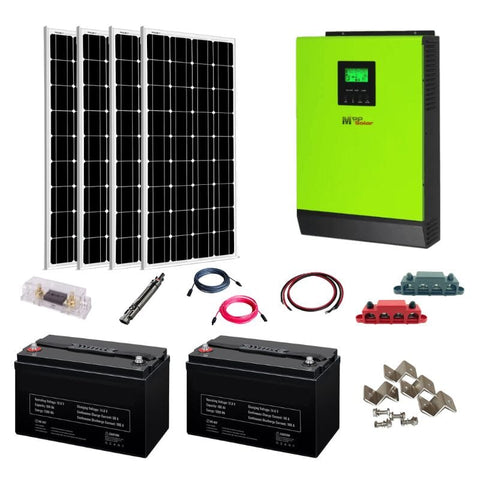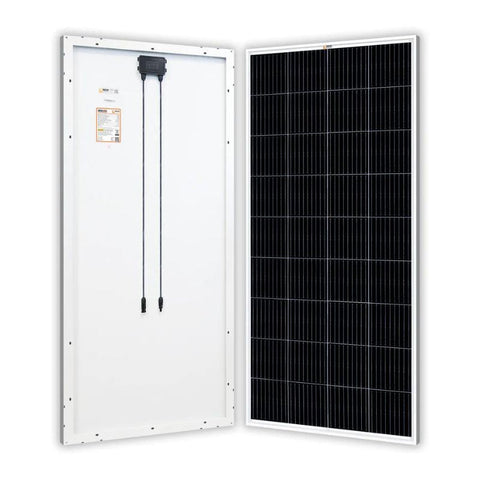Convert kW to Watts - What’s in the Conversion - ShopSolar.com

# Convert kW to Watts

Watts and kilowatts are common units in the world of solar energy.

So once we know how to convert kW to watts, we can use all sorts of other calculators that involve these units.

One example is a calculator that converts kilowatts to amps, volts, or today’s topic, watts.

So let’s unpack the process of working out watts from kW.

## The Conversion

The conversion process is relatively simple, but it relies on an understanding of the metric system.In Greek, the word ‘Kilo’ translates to ‘thousand’. This means that a kilowatt is equal to a thousand watts.

This makes the conversion easy to do in your head. To convert kW (kilowatts) to watts, we simply divide by 1,000. To do the inverse and convert watts to kilowatts, we multiply by 1,000.

For example, take a 2 kW solar system that we want to convert to watts. The maths looks like this:

W = kW x 1,000

W = 2 x 1,000

= 2 kW

Once you know your way around this, you can easily get the values you need for a kW to kWh calculator. All you need to do is work out the right value to start off.

## Watts

To understand the simple conversation of kW to watts, we’re going to look at what exactly a watt is and why it’s important in the world of solar.Watts refer to power, which is the rate that appliances use energy.

When we speak about energy, we normally use kWh as a measurement. We use this value in our energy cost per kWh calculator

For now, we’re going to focus primarily on watts and kilowatts.

### What Are Watts?

You see this unit of measurement when you look at the box of an appliance. That value displays how much power that appliance consumes.

To put this into perspective, a 100 W lightbulb is generally brighter than a 20 W bulb since the first consumes energy 5 times faster than the second.

### How to Find Watts

Ideally, if you have a value measured in kWh or MWh (megawatt-hours), you can use online calculators that convert kWh to watts.

Let’s say we want to calculate watts or use the value to obtain other units of power. You can do this by following Watt’s Law.

This law describes the relationship between power, current, and voltage in a circuit.Watts are at the top of the triangle, with current and voltage at the bottom left and right receptively.

This law is what’s used to make up the majority of online electrical calculators.

Everything from kWh to amp calculators to the larger wattage calculations used for solar off-grid systems use the same basic principles.

But let’s see how we use watts in the world of solar.

## Watts in Solar Power

Both watts and kilowatts are used in solar power systems to measure how much electricity the panels output.

In other words, the rate at which the solar system produces the electricity that we use in our homes or RV.

If we have a single 200 W flexible solar panel for an RV, that “200 W” value is how much the solar panel produces on a perfectly sunny day. The value assumes the best possible conditions, which you’re not always going to get.The same logic applies to the larger solar systems that are measured at around 5 kW. The peak power output for solar panels and systems is generally around midday.

We size solar systems using power measured in watts and kilowatts and how much power they produce under perfectly sunny conditions.

## Conclusion

In the solar world, we must understand how to convert one unit of power to another.

If we use a calculator that requires a specific unit of power and know how to obtain it, we’re left with a more accurate end value.

Did You Find Our Blog Helpful? Then Consider Checking: